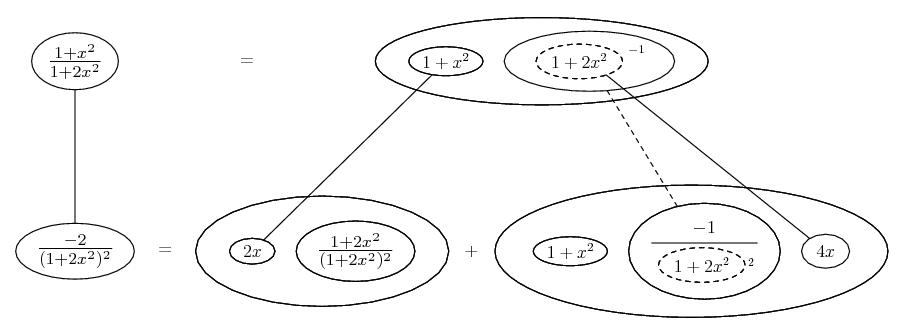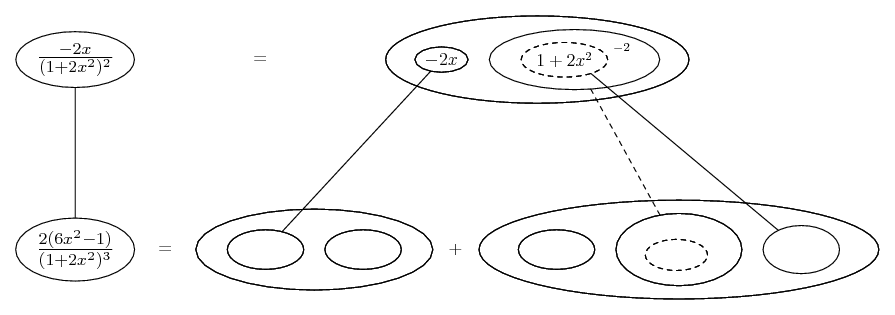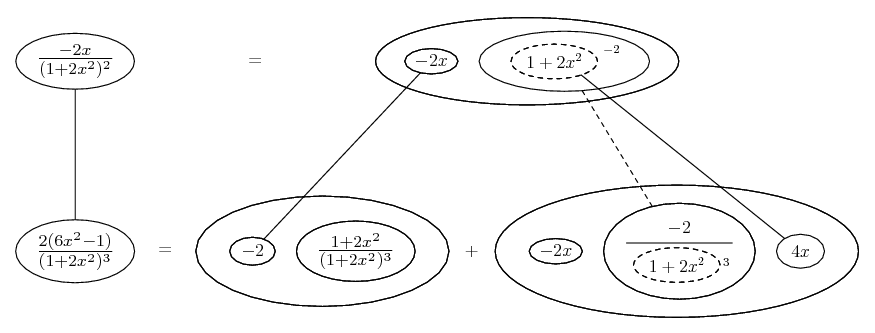# Thread: [SOLVED] 2nd differential query?

1. ##[SOLVED] 2nd differential query?

hi I'm stuck on finding the second derivative of:
y = (1+x^2)/(1+2x^2)

By hand I get:
2(8x^(2) - 1)/(2x^(2) + 1)^2 after simplifying

However, an online calculator gives me:
2(6x^(2) - 1)/(2x^(2) + 1)^2

I've checked y' but keep on getting the same answer.

Am I wrong?

Thanks, D

2. You're mis-quoting Wolfram (or whoever). The denominator is a cube not square. Check your steps and post them if not sure...

Edit:

Just in case a picture helps...... the first differentiation, where... is the product rule, and...... the chain rule, is embedded. Straight continuous lines differentiate downwards (integrate up) with respect to x, and the straight dashed line similarly but with respect to the dashed balloon expression (the inner function of the composite which is subject to the chain rule).

Then,Spoiler:_________________________________________

Don't integrate - balloontegrate!

Balloon Calculus; standard integrals, derivatives and methods

Balloon Calculus Drawing with LaTeX and Asymptote!

3. ##Yep, I see my typo. also - I wasn't expanding the top correctly prior to expanding and hence my query. Thank you

#### Search Tags

2nd, differential, query, solved